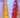## 棋子游戏

Thu, 24th October 2019Edit on Githubgamechess

medie2005提出了一个问题:

# 详细内容

s=1
s=2
s=3
s=1
s=4
s=3
s=2
s=1
s=4
s=2
s=6
s=4
s=1
s=2
s=7
s=1
s=4
s=3
s=2
s=1
s=4
s=6
s=7
s=4
s=1
s=2
s=8
s=5
s=4
s=7
s=2
s=1
s=8
s=6
s=7
s=4
s=1
s=2
s=3
s=1
s=4
s=7
s=2
s=1
s=8
s=2
s=7
s=4
s=1
s=2

O*9+E*(4+1)
12+E*(4+1)
O*5+O*(4+1)
E*5+A*(4+1)
A*20+E*(17+12+9)+A*(4+1)
25+O*(20+4+1)+E*(17+12+9)
25+O*9+O*(4+1)

Github